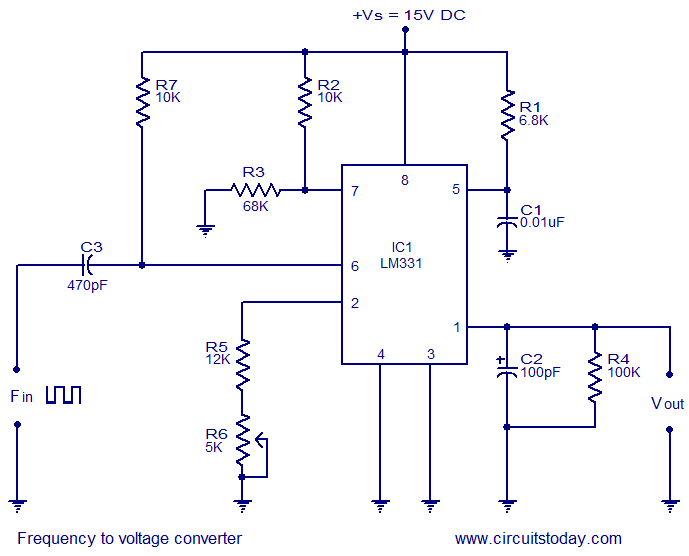## Audio

[Audio][slideshow]

Description.
LM331 is basically a precision voltage to frequency converter from National Semiconductors. The IC has a hand full of applications like analog to digital conversion, long term integration, voltage to frequency conversion, frequency to voltage conversion. Wide dynamic range and excellent linearity makes the IC well suitable for the applications mentioned above.
Here the LM331 is wired as a frequency to voltage converter which converts the input frequency into a proportional voltage which is extremely linear to the input frequency. The frequency to voltage conversion is attained by differentiating the input frequency using capacitor C3 and resistor R7 and feeding the resultant pulse train to the pin6 (threshold) of the IC. The negative going edge of the resultant pulse train at pin6 makes the built-in comparator circuit to trigger the timer circuit. At any instant, the current flowing out of the current output pin (pin 6) will be proportional to the input frequency and value of the timing components (R1 and C1). As a result a voltage (Vout) proportional to the input frequency (Fin) will be available across the load resistor R4.

Circuit diagram.Notes.

• The circuit can be assembled on a vero board.
• I used 15V DC as the supply voltage (+Vs) while testing the circuit.
• The LM331 can be operated from anything between 5 to 30V DC.
• The value of R3 depends on the supply voltage and the equation is R3= (Vs – 2V)/ (2mA).
• According to the equation, for Vs = 15V, R3=68K.
• The output voltage depends on the equation, Vout = ((R4)/(R5+R6))*R1C1*2.09V*Fin.
• POT R6 can be used for calibrating the circuit.

[Lights][grids]